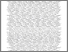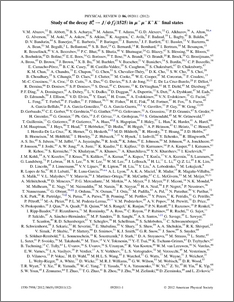# Study of the decay $B_s^0 \rightarrow J/ψf_2^{\prime}(1525)$ in $μ^+ μ^- K^+K^-$ final states

Collaboration, D0 and Bertram, Iain and Borissov, Guennadi and Fox, Harald and Ross, Anthony and Williams, Mark and Ratoff, Peter (2012) Study of the decay $B_s^0 \rightarrow J/ψf_2^{\prime}(1525)$ in $μ^+ μ^- K^+K^-$ final states. Physical Review D, 86 (9). ISSN 1550-7998Preview
PDF
PhysRevD.86.092011.pdf - Submitted Version

## Abstract

We investigate the decay $B_s^0 \rightarrow J/\psi K^+ K^-$ for invariant masses of the $K^+K^-$ pair in the range \$1.35M(K^+K^-)

Item Type:
Journal Article
Journal or Publication Title:
Physical Review D